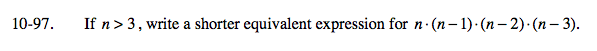### Home > A2C > Chapter 10 > Lesson 10.2.3 > Problem10-97

10-97.

If n > 3, write a shorter equivalent expression for n (n − 1)(n − 2)(n − 3). Homework Help ✎Use factorial form. Think of which factors will cancel.

$\frac{n!}{(n-4)!}$